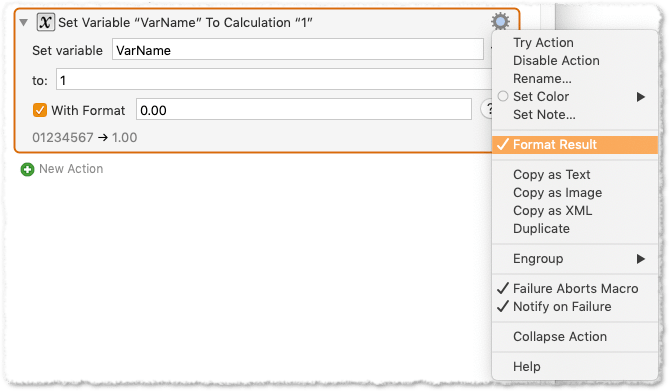Hi!

Beginner help appreciated.

I am manipulation numeric data that is normally to three decimal places (it is consistently never more). However, if the last digit is a '0', the source does not output the last 'zero'.
So for example I would have
1.127
1.128
1.129
1.13 <<<<
1.131

I assume that I can use the 'character count' filter' to identify any numbers with 'two decimal point digits' (the numbers of characters before the decimal place is consistently a single digit.)
But how do I append a '0' to the end of any number that has just two decimal places?

Help appreciated.

Thanks

Mik

Not sure if that’s what you are looking for, but in calculation actions you can set the format:PS:

Select “Help” in the gear menu to find further explanations.

1 Like

I use the latter in this example Macro:
`%CalculateFormat%Local__Number%0.000%`

### Example Output### MACRO:   Zero Fill Decimal Places [Example]

~~~ VER: 1.0    2019-07-19 ~~~